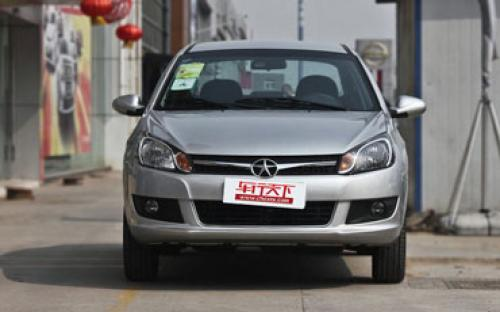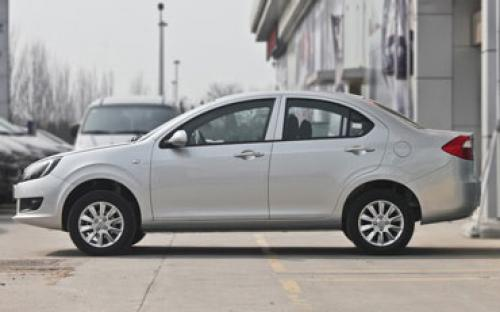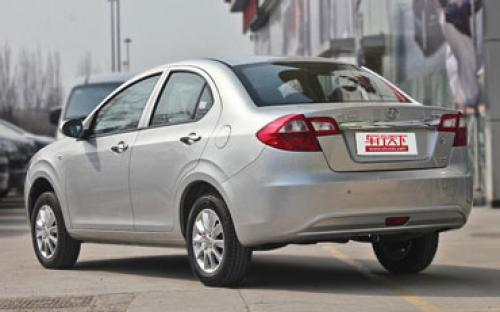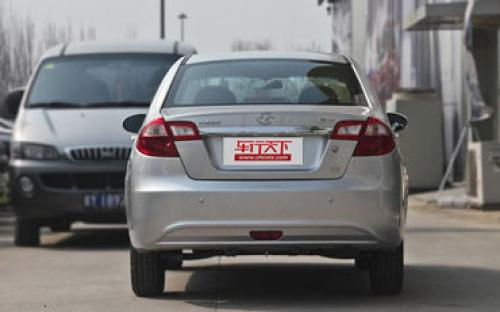江淮 同悦8 种颜色可选2012款最低售价：4.88 万元起

4155(mm)1650(mm)1445(mm)配置亮点：
• 胎压监测装置

• ISOFIX儿童座椅接口

• 车身稳定控制(ESC/ESP/DSC等)

• 电动天窗

• 定速巡航

• 后倒车雷达

• 真皮座椅

• GPS导航系统

• 氙气大灯

• 后视镜加热江淮 同悦 在售车型

排量 车型 厂商指导价 本地最低报价 购车工具
1.3L 1.3L 手动标准型 停产停售 5挡手动 4.88万 4.88万

询底价+对比
1.3L 手动舒适型 停产停售 5挡手动 5.18万 5.18万

询底价+对比
1.3L 手动豪华型 停产停售 5挡手动 5.38万 5.38万

询底价+对比
1.3L AMT豪华型 停产停售 5挡AMT 5.68万 5.68万

询底价+对比

江淮 同悦 经销商

查看更多 >>
更多 >>

本地降价排名

江淮 同悦 动力加速

同悦 0-100公里加速时间分布在 0.0-秒 属于 超跑级

动力级别 加速时间 车型

江淮 同悦 视频

江淮 同悦 新闻资讯

将申报召回同悦小型车 江淮官方声明

技术 超过5928次关注

今日，江淮汽车发表官方声明，经过研究，江淮决定就已销售的同悦轿车车身锈蚀问题向国家质检总局缺陷产品管理中心（召回中心）申报召回。

同悦满月照暨购车作业

车系文章 超过2684次关注

第一章：序曲2011年10月12日驾照到手。其实，我虽然也有学驾驶的想法，但仅仅是想学会了可以临时开车玩玩，根本就没有学会了去买车的

1.5L自主品牌三厢家轿导购 便宜又实用

车系文章 超过3298次关注

经济危机之后中国车市当中有一个现象，1.5L排量的发动机似乎越来越受到自主品牌厂商们的青睐，不仅仅传统车型加大了1.5L车型的比重，更多的新车型更是将这一排量作...

测试2010款同悦1.3L VVT 性能有所提升

车系文章 超过2643次关注

2010年，又有不少车型推出了改款，江淮同悦就是其中之一，不过它可没有将外观作为主要的改动部分，可以这样说，如果你不仔细观察的话是很难看出差别的，当然如果你...

江淮官方表示 同悦小型车将被申报召回

车系文章 超过2015次关注

江淮汽车发表官方声明，经过研究，江淮决定就已销售的同悦轿车车身锈蚀问题向国家质检总局缺陷产品管理中心（召回中心）申报召回。

同悦购车享补贴再送港澳游和装饰 部分现车

车系文章 超过1839次关注

近日，在河南世纪阳光汽车销售服务有限公司了解到，目前店内同悦全系只有手动豪华型有现车，其他车型需预订，购车享3000元补贴，再送2000元装饰和双人4日港澳游。

北京车展探馆：江淮改款同悦车型曝光

车系文章 超过2036次关注

在北京车展前的探馆工作中，我们在江淮展台看见了改款同悦的身影，而该车将在本届车展中正式发布。改款同悦除了改变了外观和内饰之外，其车身尺寸也做了调整。

猜你喜欢

﻿
• 快速找车
• 选择品牌
• 选择品牌
• A  奥迪
• A  阿斯顿·马丁
• A  阿尔法·罗密欧
• B  宝沃
• B  布加迪
• B  巴博斯
• B  保时捷
• B  宾利
• B  奔驰
• B  宝马
• B  本田
• B  别克
• B  标致
• B  比亚迪
• B  宝骏
• B  北汽制造
• B  北汽新能源
• B  北汽幻速
• B  北汽威旺
• B  北京汽车
• B  奔腾
• B  北汽绅宝
• C  长安
• C  长安商用
• C  长城
• C  昌河
• D  大众
• D  道奇
• D  DS
• D  东南
• D  东风风神
• D  东风风行
• D  东风小康
• D  东风风度
• D  东风
• F  福特
• F  丰田
• F  菲亚特
• F  法拉利
• F  福田
• F  福迪
• F  福汽启腾
• G  观致
• G  广汽传祺
• G  广汽吉奥
• G  GMC
• H  红旗
• H  汉腾汽车
• H  哈弗
• H  哈飞
• H  海格
• H  海马
• H  华颂
• H  黄海
• H  华泰
• H  恒天
• J  吉利汽车
• J  捷豹
• J  Jeep
• J  江淮
• J  江铃
• J  金杯
• J  九龙
• J  金旅
• K  凯翼
• K  凯迪拉克
• K  克莱斯勒
• K  科尼塞克
• K  卡威
• K  开瑞
• L  路虎
• L  林肯
• L  劳斯莱斯
• L  兰博基尼
• L  雷克萨斯
• L  铃木
• L  雷诺
• L  理念
• L  力帆
• L  莲花汽车
• L  猎豹
• L  路特斯
• L  陆风
• M  马自达
• M  MG
• M  MINI
• M  玛莎拉蒂
• M  摩根
• M  迈凯轮
• N  纳智捷
• O  欧宝
• O  讴歌
• O  欧朗
• Q  奇瑞
• Q  起亚
• Q  启辰
• R  日产
• R  荣威
• R  瑞麒
• S  三菱
• S  斯威汽车
• S  萨博
• S  smart
• S  斯柯达
• S  斯巴鲁
• S  思铭
• S  双龙
• S  上汽大通
• S  双环
• T  特斯拉
• T  腾势
• W  沃尔沃
• W  五菱汽车
• W  五十铃
• W  威兹曼
• W  威麟
• X  现代
• X  雪佛兰
• X  雪铁龙
• X  西雅特
• Y  一汽
• Y  英菲尼迪
• Y  英致
• Y  依维柯
• Y  野马汽车
• Y  永源
• Z  众泰
• Z  中华
• Z  中兴
• Z  知豆
• 选择车系
• 选择车系
• 车型对比
• 选择品牌
• 选择品牌
• A  奥迪
• A  阿斯顿·马丁
• A  阿尔法·罗密欧
• B  宝沃
• B  布加迪
• B  巴博斯
• B  保时捷
• B  宾利
• B  奔驰
• B  宝马
• B  本田
• B  别克
• B  标致
• B  比亚迪
• B  宝骏
• B  北汽制造
• B  北汽新能源
• B  北汽幻速
• B  北汽威旺
• B  北京汽车
• B  奔腾
• B  北汽绅宝
• C  长安
• C  长安商用
• C  长城
• C  昌河
• D  大众
• D  道奇
• D  DS
• D  东南
• D  东风风神
• D  东风风行
• D  东风小康
• D  东风风度
• D  东风
• F  福特
• F  丰田
• F  菲亚特
• F  法拉利
• F  福田
• F  福迪
• F  福汽启腾
• G  观致
• G  广汽传祺
• G  广汽吉奥
• G  GMC
• H  红旗
• H  汉腾汽车
• H  哈弗
• H  哈飞
• H  海格
• H  海马
• H  华颂
• H  黄海
• H  华泰
• H  恒天
• J  吉利汽车
• J  捷豹
• J  Jeep
• J  江淮
• J  江铃
• J  金杯
• J  九龙
• J  金旅
• K  凯翼
• K  凯迪拉克
• K  克莱斯勒
• K  科尼塞克
• K  卡威
• K  开瑞
• L  路虎
• L  林肯
• L  劳斯莱斯
• L  兰博基尼
• L  雷克萨斯
• L  铃木
• L  雷诺
• L  理念
• L  力帆
• L  莲花汽车
• L  猎豹
• L  路特斯
• L  陆风
• M  马自达
• M  MG
• M  MINI
• M  玛莎拉蒂
• M  摩根
• M  迈凯轮
• N  纳智捷
• O  欧宝
• O  讴歌
• O  欧朗
• Q  奇瑞
• Q  起亚
• Q  启辰
• R  日产
• R  荣威
• R  瑞麒
• S  三菱
• S  斯威汽车
• S  萨博
• S  smart
• S  斯柯达
• S  斯巴鲁
• S  思铭
• S  双龙
• S  上汽大通
• S  双环
• T  特斯拉
• T  腾势
• W  沃尔沃
• W  五菱汽车
• W  五十铃
• W  威兹曼
• W  威麟
• X  现代
• X  雪佛兰
• X  雪铁龙
• X  西雅特
• Y  一汽
• Y  英菲尼迪
• Y  英致
• Y  依维柯
• Y  野马汽车
• Y  永源
• Z  众泰
• Z  中华
• Z  中兴
• Z  知豆
• 选择车系
• 选择车系
• 选择车型
• 选择车型
• 意见反馈